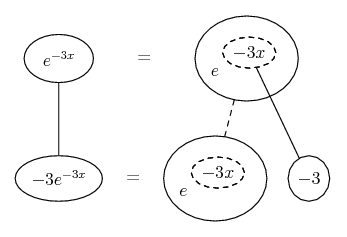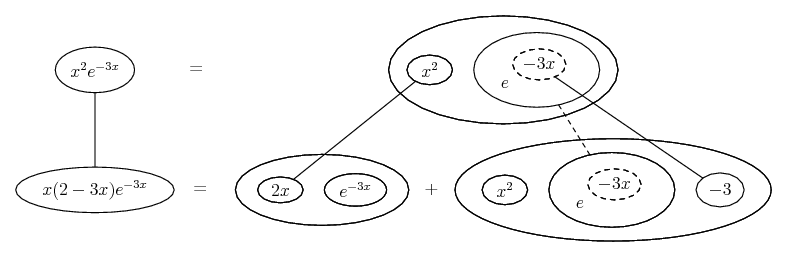# Thread: Derivate f(x)=x^2*e*(-3x)

1. ## Derivate f(x)=x^2*e*(-3x)

Hi guys

I've been trying to solve this for a little while now but I feel kinda lost and I thought you could maybe help me out.

so

f(x)=x^2*e^(-3x)

f '(x)=?

Thank you.

2.Originally Posted by BlacklawHi guys

I've been trying to solve this for a little while now but I feel kinda lost and I thought you could maybe help me out.

so

f(x)=x^2*e^(-3x)

f '(x)=?

Thank you.
$\displaystyle f(x) = g(x)h(x)$, then $\displaystyle f'(x) = g'(x)h(x) + g(x)h'(x)$

take $\displaystyle g(x) = x^2$ and $\displaystyle h(x) = e^{-3x}$

3.Originally Posted by dedust$\displaystyle f(x) = g(x)h(x)$, then $\displaystyle f'(x) = g'(x)h(x) + g(x)h'(x)$

take $\displaystyle g(x) = x^2$ and $\displaystyle h(x) = e^{-3x}$
I get $\displaystyle 2x*e^{-3x}+x^2*e^{-3x}$

But I know for a fact that the correct answer is $\displaystyle 2x*e^{-3x}+x^2*e^{-3x}*(-3)$

Please explain how I get the (-3)?

4.Originally Posted by BlacklawI get $\displaystyle 2x*e^{-3x}+x^2*e^{-3x}$

But I know for a fact that the correct answer is $\displaystyle 2x*e^{-3x}+x^2*e^{-3x}*(-3)$

Please explain how I get the (-3)?
$\displaystyle \frac{d}{dx}e^{-3x}=-3e^{-3x}$

You did not know the answer for a fact if it was what was in the back of the book. You know it for a fact if you know why it is so and now you should do.

CB

5.Originally Posted by CaptainBlack$\displaystyle \frac{d}{dx}e^{-3x}=-3e^{-3x}$

You did not know the answer for a fact if it was what was in the back of the book. You know it for a fact if you know why it is so and now you should do.

CB
Does it always go like that? I thought the derivative of $\displaystyle e^x$ was $\displaystyle e^x$?

Thank you for you help, I truly appreciate it.

6.Originally Posted by BlacklawDoes it always go like that?
That's what the chain rule says.

Just in case a picture helps...is the chain rule. Straight continuous lines differentiate downwards (integrate up) with respect to x, and the straight dashed line similarly but with respect to the dashed balloon expression (the inner function of the composite which is subject to the chain rule).

In this case...PS:

Just in case another picture doesn't hurt (and in view of comments below)...

Wrapping the chain rule inside the (legs-uncrossed version of) ...... the product rule, we get...__________________________________________
Don't integrate - balloontegrate!

Balloon Calculus; standard integrals, derivatives and methods

Balloon Calculus Drawing with LaTeX and Asymptote!

7. I think I get it now.. Thank you very much for helping me out.

8. $\displaystyle f(x) = x^2 e^{-3x}$
$\displaystyle f'(x) = x^2 e^{-3x} (-3) + e^{-3x} 2x$ By chain rule
$\displaystyle f'(x) = x e^{-3x} [ -3x + 2 ]$

Hope this helps.

9.Originally Posted by rshekhar.in$\displaystyle f(x) = x^2 e^{-3x}$
$\displaystyle f'(x) = x^2 e^{-3x} (-3) + e^{-3x} 2x$ By chain rule
$\displaystyle f'(x) = x e^{-3x} [ -3x + 2 ]$

Hope this helps.
What you label as "by chain riue" is mainly product rule and to a lesser extent chain rule

CB

10.Originally Posted by BlacklawDoes it always go like that? I thought the derivative of $\displaystyle e^x$ was $\displaystyle e^x$?

Thank you for you help, I truly appreciate it.
The derivative of $\displaystyle e^x$ is $\displaystyle e^x$ but that is not what you have, what you should know is that the derivative of $\displaystyle e^{ax}$ is $\displaystyle ae^{ax}$ by the chain rule if you must, but you should know it because it will occur so often.

CB

#### Search Tags

derivate, fxx2e3x